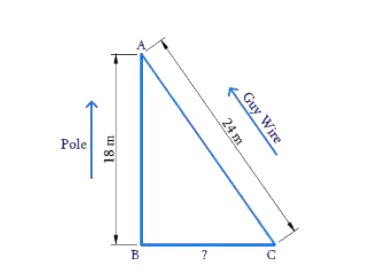# Ex.6.5 Q10 Triangles Solution - NCERT Maths Class 10

Go back to  'Ex.6.5'

## Question

A guy wire attached to a vertical pole of height $$18$$$$\rm{}m$$ is $$24$$ $$\rm{}m$$ long and has a stake attached to the other end. How far from the base of the pole should the stake be driven so that the wire will be taut?

Diagram## Text Solution

Reasoning:

In a right triangle, the square of the hypotenuse is equal to the sum of the squares of the other two sides.

Steps:

$$AB$$ is the length of the pole = $$18$$$$\rm{}m$$

$$AC$$ is the length of the guy wire = $$24$$$$\rm{}m$$

$$BC$$ is the distance of the stake from the pole $$= ?$$

In$$\Delta ABC\,\,\,\,\,\angle ABC={{90}^{0}}$$

\begin{align} B C^{2} &=A C^{2}-A B^{2} \text { (Pythagoras theorem) } \\ B C^{2}&=24^{2}-18^{2} \\ B C^{2}&=576-324 \\ B C^{2}&=252 \\ B C &=2 \times 3 \sqrt{7} \\ B C &=6 \sqrt{7} \end{align}

The distance of the stake from the pole $$=6\sqrt{7}\rm{}m$$

Learn from the best math teachers and top your exams

• Live one on one classroom and doubt clearing
• Practice worksheets in and after class for conceptual clarity
• Personalized curriculum to keep up with school